Science, Maths & Technology

### Become an OU studentAn introduction to exoplanets

Start this free course now. Just create an account and sign in. Enrol and complete the course for a free statement of participation or digital badge if available.

# 2.4  Circles and spheres

Once the size of a star is known, it is possible to work out the size of any planet that transits in front of it. This is done simply by measuring how much light from the star is blocked by the planet.

But how much light is that? To work it out, you need to think about the cross-sectional area of a sphere.

## Activity _unit5.2.1 Activity 1  Cross-sectional shapes

The cross-section of an object is the shape you would see if you took a slice through that object. Can you work out the cross-sectional shapes of these solids?

1. What shape is the cross-section of this cube?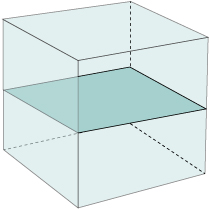Figure _unit5.2.6 Figure 8  Cube with cross-section cut marked

a.

Square

b.

Diamond

c.

Rectangle

2. What shape is the cross-section of this cone?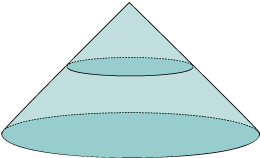Figure _unit5.2.7 Figure 9  Cone with cross-section cut marked

a.

Triangle

b.

Circle

c.

Square

3. What shape is the cross-section of this solid?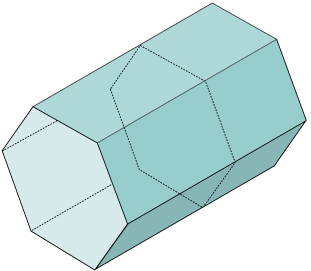Figure _unit5.2.8 Figure 10  Prism with cross-section cut marked

a.

Square

b.

Diamond

c.

Hexagon

In terms of transits, it is important to know what a sphere looks like if it’s projected onto a flat surface. When we view spherical objects such as footballs and planets we see their cross-section, which is a circle.

The term ‘cross-sectional area’ refers to the area of the shape that is the cross-section. So, the cross-sectional area of a sphere is the area of the circle we see. The radius of this circle, the distance from the centre to the edge, is just the same as the radius of the sphere (Figure 11).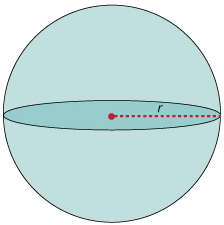Figure _unit5.2.9 Figure 11  A circle as a cross-section of a sphere of radius r

As with the pictures of the transits of Mercury and Venus in Figures 3 and 4, if you were close to the host star of a transiting exoplanet, you would see a bright circle of star with a smaller dark circle of planet on top of it. To work out how much of the bright star is ‘missing’, you need to know the areas of these two circles.

The host stars are incredibly far away though, so we can’t see these circles – all we can measure is the amount of light coming from the point of light that is the star. Figure 12 shows how the light coming from a star is reduced when a planet transits in front of it. This graph measuring the amount of light, with its particular shape, is called a light curve. The dip in the light curve tells us how much of the star’s light is blocked by the planet, from which we can calculate the relative areas of the two circles and hence the size of the planet.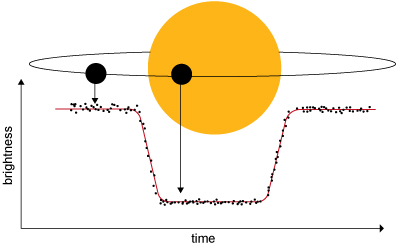Figure _unit5.2.10 Figure 12  Schematic of a transit and the measured light curve